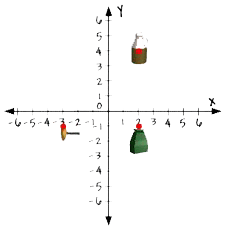Home    |    Teacher    |    Parents    |    Glossary    |    About UsYou used the coordinate plane in algebra for graphing and solving equations. This powerful tool is also used in studying geometry. Although the idea of graphing on a coordinate plane dates all the way back to the 1600's, it didn't appear in our high school math textbooks until the early 1900's. Since that time it's become more and more important for solving different types of math problems.

We can graph lines, line segments, rays, and angles—as well as other geometric figures—on the coordinate plane and use the graphs to help us determine their relationship to each other as well as other features such as length.

Here's one way geometry is used in the real world. A team of archaeologists is studying the ruins of Lignite, a small mining town from the 1800's. They plot points on a coordinate plane to show exactly where each artifact is found.They are using this coordinate plane as a map of a section of the town. It shows the location of a medicine bottle, a doorknob, and a pottery jug. Notice that each unit on the grid is equal to 5 meters. How far apart are the doorknob and the medicine bottle? The doorknob is at point (-3, -1) and the medicine bottle is at point (2, -1).

If we count the units between the two points, we find that they are 5 units apart. We could also subtract the x coordinates of the two endpoints of the line segment to find the distance between them: "two minus negative 3 is equal to five." Since each unit is equal to 5 meters, we multiply the 5 units times 5 meters. The two artifacts are 25 meters apart.

This example shows just one way that geometry can help people to solve real world problems. It's also used in building, in art, in clothing design and manufacture, in landscaping, in exploring space, and in organizing closets—in fact, there are so many uses for geometry that we could never name them all!

 Homework Help | Geometry | Geometry Building BlocksEmail this page to a friendSearch·  Words·  Coordinate geometry·  Pairs of lines·  Classifying angles·  Angles and     intersecting lines·  CirclesFirst Glance In Depth Examples WorkoutCoordinate geometry